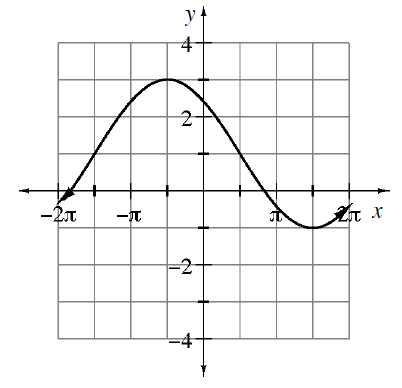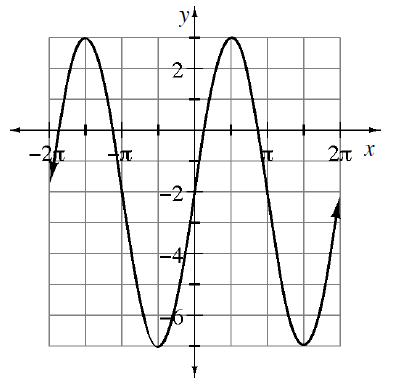### Home > INT3 > Chapter Ch12 > Lesson 12.1.4 > Problem12-67

12-67.

Write a possible equation for the graphs shown below. Then state the amplitude and period of the function. Describe how the graph relates to the parent function. Homework Help ✎

Look at the graph to determine the amplitude, period, phase shift, and vertical shift.

1. parent: $y=\sin(x)$Use the information in $y = a\ \text{sin}\ b\left(x + c\right) + d$

$y=-2 \text{ sin}\left (\frac{1}{2}\left (x-\frac{\pi}{2}\right )\right )+1$

1. parent: $y=\cos(x)$